# Test: Class 12 Physics: CBSE Sample Question Paper- Term I (2021-22)

## 55 Questions MCQ Test Sample Papers for Class 12 Medical and Non-Medical | Test: Class 12 Physics: CBSE Sample Question Paper- Term I (2021-22)

Description
Attempt Test: Class 12 Physics: CBSE Sample Question Paper- Term I (2021-22) | 55 questions in 90 minutes | Mock test for Class 12 preparation | Free important questions MCQ to study Sample Papers for Class 12 Medical and Non-Medical for Class 12 Exam | Download free PDF with solutions
QUESTION: 1

### Which of the following is NOT the property of equipotential surface?

Solution:

As all other statements are correct. In uniform electric field, equipotential surfaces are never concentric spheres but are planes ⊥ to electric field lines.

QUESTION: 2

### Two point charges + 8q and –2q are located at x = 0 and x = L respectively. The point on x axis at which net electric field is zero due to these charges is

Solution:

Let P be the observation point at a distance r from –2q and at (L + r) from + 8q.
Given Now, Net EFI at P = 0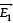= EFI (Electric Field Intensity) at P due to + 8q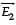= EFI (Electric Field Intensity) at P due to – 2q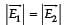∴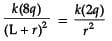∴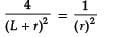4r2 = (L + r)2
2r = L + r
r = L
∴ P is at x = L + L = 2L from origin
∴ Correct option is (C) 2L.

QUESTION: 3

### An electric dipole of moment p is placed parallel to the uniform electric field. The amount of work done in rotating the dipole by 90° is

Solution:

W = pE (cos θ1 – cos θ2)
θ1 = 0°
θ2 = 90°
W = pE (cos 0° – cos 90°)
= pE (1 – 0)
= PE

QUESTION: 4

hree capacitors 2 µF, 3 µF and 6 µF are joined in series with each other.
The equivalent capacitance is:

Solution: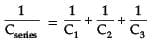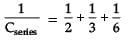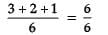Cseries = 1 µF

QUESTION: 5

Two point charges placed in a medium of dielectric constant 5 are at a distance r between them, experience an electrostatic force ‘F’. The electrostatic force between them in vacuum at the same distance r will be:

Solution: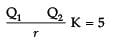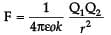Q1 _______Q2
Force in the charges in the air is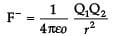= K F
= 5 F

QUESTION: 6

Which statement is true for Gauss law?

Solution:

All other statements except (iv) are incorrect.
The electric field over the Gaussian surface remains continuous and uniform at every point.

QUESTION: 7

A capacitor plates are charged by a battery with ‘V’ volts. After charging, battery is disconnected and a dielectric slab with dielectric constant ‘K’ is inserted between its plates, the potential across the plates of a capacitor will become:

Solution: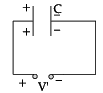Battery is disconnected.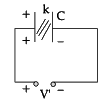Q = Charge remains context C′ = KC
Q′ = C′V′
Q = C′V′
Q = KCV′

V′ =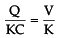QUESTION: 8

The best instrument for accurate measurement of EMF of a cell is

Solution:

Potentiometer

QUESTION: 9

An electric current is passed through a circuit containing two wires of same material, connected in parallel. If the lengths and radii of the wires are in the ratio of 3 : 2 and 2 : 3, then the ratio of the current passing through the wire will be

Solution: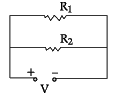I1 : I2 = 3 : 2
r1 : r2 = 2 : 3
I1 : I2 = ?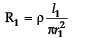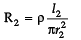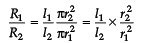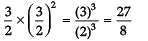∴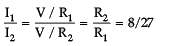QUESTION: 10

By increasing the temperature, the specific resistance of a conductor and a semiconductor:

Solution:

Specific resistance of a conductor increases and for a semiconductor decreases with increase in temperature because for a conductor, a temperature:
coefficient of resistivity, a = + ve
and for a semiconductor, a = – ve

QUESTION: 11

We use alloys for making standard resistors because they have:

Solution:

Alloys have low temperature coefficient of resistivity and high specific resistance. If a = low, the value of ‘R’ with temperature will not change much and specific resistance is high, then required length of the wire will be less.

QUESTION: 12

A constant voltage is applied between the two ends of a uniform metallic wire, heat ‘H’ is developed in it. If another wire of the same material, double the radius and twice the length as compared to original wire is used, then the heat developed in it will be:

Solution: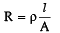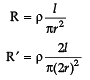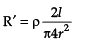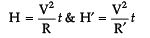∵ V = constant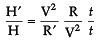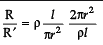H′ /H = 2/1
H′ = 2H

QUESTION: 13

If the potential difference V applied across a conductor is increased to 2V with its temperature kept constant, the drift velocity of the free electrons in a conductor will:

Solution:

We know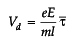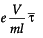If temperature is kept constant, relaxation time τ - will remain constant and e, m are also constants.
Vd ∝ V
Vd ∝ 2V

QUESTION: 14

The SI unit of magnetic field intensity is:

Solution:

We know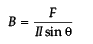SI Unit of B = N/Am = NA1m–1

QUESTION: 15

The equivalent resistance between A and B is:

Solution:

Redrawing the circuit, we get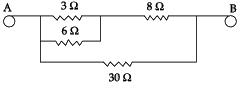3 Ω & 6 Ω are in parallel.
∴ R1 =  3 x 6 / 3 + 6 = 18/9 = 2

Now R1 and 8Ω in series
∴ R2 = R1 + 8 = 2 + 8 = 10 Ω
Now R2 and 30Ωin parallel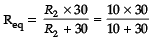= 300/40 = 30/4 = 15 /2
= 7.5 Ω

QUESTION: 16

Two wires of the same length are shaped into a square of side 'a' and a circle with radius 'r'. If they carry same current, the ratio of their magnetic moment is:

Solution:

l = length of wire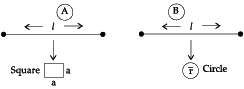Area of a Square = a2
Also here l = 4a
a = l /4
∴ Area = l2 /16
A1 = l2/16
Area of a circle = πr2
Also here, 2πr = l
r = l/2π
Now area =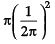A2 = l2/4π
Now Magnetic moment = IA
∴ M1 = IA, & M2 = I A2
Since I (current) is same in both
∴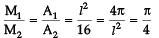M1M2 = π : 4

QUESTION: 17

The coil of a moving coil galvanometer is wound over a metal frame in order to:

Solution:

The coil of a moving coil galvanometer is wound over metallic frame to provide electromagnetic damping so it becomes dead beat galvanometer.

QUESTION: 18

The small angle between magnetic axis and geographic axis at a place is:

Solution:

Correct option is Magnetic declination or Angle of declination. It is the small angle between geographic axis and magnetic axis.

QUESTION: 19

The horizontal component of earth’s magnetic field at a place is 3 times the vertical component. The angle of dip at that place is:

Solution:

Target law, BV = BH tanδ
tanδ = BV / BH
Given BH = √3BV
tanδ = BV / √3BV = 1/√3
δ = 30° or π / 6 radians.

QUESTION: 20

A conducting square loop of side 'L' and resistance 'R' moves in its plane with the uniform velocity 'v' perpendicular to one of its sides. A magnetic induction 'B' constant in time and space pointing perpendicular and into the plane of the loop exists everywhere as shown in the figure. The current induced in the loop is: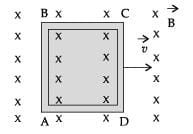Solution:

Current induced is I = |e/R|

Now |e| = dφ/dt
But there is no change of flux with time, as B',A' and θ all remain constant with time.
∴ No current is induced.

QUESTION: 21

Two coils are placed close to each other. The mutual inductance of the pair of coils depends upon the:

Solution:

Mutual inductance of a pair of two coils depends on the relative position and orientation of two coils.

QUESTION: 22

Which of the following graphs represent the variation of current(I) with frequency (f) in an AC circuit containing a pure capacitor?

Solution:

I = V / X in pure capacitor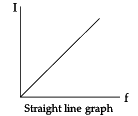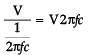QUESTION: 23

The magnetic flux linked with the coil (in Weber) is given by the equation:
φ = 5t2 + 3t + 16 The induced EMF in the coil at time, t = 4 will be:

Solution:

φ = 5t2 + 3t + 16
|e| = dφ/dt
= d/dt(5t2 + 3t + 16)
= 10t + 3
|e|t=4 = 10(4) + 3 = 43 V
e = - 43 Volts

QUESTION: 24

The instantaneous values of emf and the current in a series ac circuit are: E = Eo sin ωt and I = Io sin (ωt + π/3) respectively, then it is

Solution:

E = Eo sin ωt
I =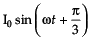as I can lead the voltage in RC and LCR circuit, so it can be RC or LCR circuit.

QUESTION: 25

A 20 volt AC is applied to a circuit consisting of a resistance and a coil with negligible resistance. If the voltage across the resistance is 12 volt, the voltage across the coil is:

Solution: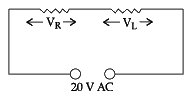VR = Effective voltage across R
∴ VR = Ieff R
VL = Effective voltage across L
VL = Ieff × L
Net V =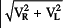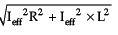20 =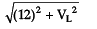(20)= (12)2 + VL2
400 = 144 + VL2
VL = √400 - 144 = √266 = 16 volts

QUESTION: 26

Electrostatic force between two charged particles in air is F. If charges are placed at the same distance in brass, what will be the force?

Solution:

Since, dielectric constant of metal is ∞. The electric force between the charges in any medium is given by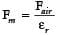⇒ Fm = F/∞
⇒ Fm = 0

QUESTION: 27

If the electric field is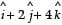. Electric flux through a surface area 10 units lying in X-Y plane is

Solution:

Direction of area element is normal to X-Y plane, i.e., z axis. Therefore,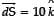. Now, using the relationship between electric flux f and electric field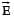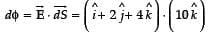= 40 units

QUESTION: 28

Work done in moving an electron from one point to another on an equipotential surface of 10 Volt potential

Solution:

The potential difference between two points of equipotential surface i.e.
V2 - V1 = 0 = W/q ⇒ W = 0
Therefore, no work done is done in moving a charge from one point to another on an equipotential surface.

QUESTION: 29

Electric potential at a point (x, y, z) in vacuum is V = 3x2 volt. Find the electric field intensity at the point (1m, 2m, 3m) .

Solution:

We know that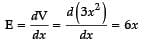Here x = 1 m
Hence, E = 6 V/m.

QUESTION: 30

Two copper wires of same length, having different cross-sectional area in the ratio 1:3, are connected in series. The ratio of their drift velocities vd will be

Solution:

Since, two copper wires are connected in series, current i flow through them is same. Both wires are made of same material, so n1 = n2
As, i = neAvd
⇒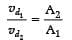⇒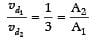⇒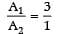QUESTION: 31

When A wire of resistance 12 R is bent to form a circle. The effective resistance across its diameter is

Solution:

Given that resistance of wire 12 Ω. It is bent and form circle, then resistance f each semicircle is 6 Ω. Now, measuring the resistance across its diameter, these two resistances are in parallel. Their effective resistance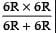=3R

QUESTION: 32

Two heaters A (500 W, 220 V) and B (1000 W, 220 V) are connected in parallel to 220 V. Which heater produces more heat

Solution:

As P = V x i = i2 R = V2/R
R for heater A

RA = V2/P = (200)2 /500 = 96.8Ω
R for heater B
RB = V2/P = (200)2 /1000 = 48.4Ω
⇒ RA >RB
When two heaters are connected in parallel, voltage remains same for two resisters and power is calculated using the following formula:
P = V2/R
⇒ PA <PB
Therefore, power dissipation is small in heater A as compare to heater B.
Heat generated H = P × t, where t is time in seconds.
⇒ HA <HB

QUESTION: 33

When two bulbs A (40 W, 220 V) and B (60 W, 220 V) are connected in series, which bulb is brighter.

Solution:

As
P = V x i = i2R = V2/R
R for 40 W bulb
R40 = V2/P = (200)2/40 = 1210Ω
R for 60 W bulb
R60 = V2/P = (200)2/60 = 806.67Ω
⇒ R40>R60
When two bulbs are connected in series, current flow through them is same and power is calculated using the following formula:
P = i2R or P  ∞ R
Since, R40 > R60 therefore, P40 > P60 Therefore, power dissipation is large in 40 W bulb as compare to 60 W bulb. 40 W bulb is brighter than 60 W.

QUESTION: 34

The magnetic field due to a current carrying circular loop of radius 3 cm at a point on the axis at a distance of 4 cm from the center is 54 mT. What will be its value at the centre of the loop?

Solution:

Magnetic field due to current carrying circular loop of radius a at a point on the axis at a distance x from the centre is given by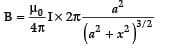⇒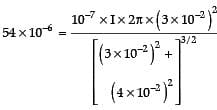⇒ I  = 11.94 A
Magnetic field at the centre =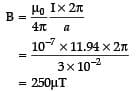QUESTION: 35

A current i ampere flows along an infinite long straight tube, then the magnetic induction at any point inside

Solution:

Using Ampere’s law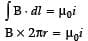Here, i is zero, for r < R, where R is radius of the tube
∴   B = 0

QUESTION: 36

A long solenoid is formed by 70 turns/cm. The current to produce a magnetic field of 20 mT inside the solenoid will be

Solution:

B = μ0ni
⇒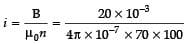= 2.27A

QUESTION: 37

A charged particles enters into a magnetic field with an angle of 30° with respect to the direction of magnetic field. The path of the particle is

Solution:

Charge is entered at point A making an angle 30°with the velocity v. one component of it is along the field and other component is perpendicular to it. Its perpendicular component v sin 30° will create circular motion, whereas its component along the field v cos 30° will have linear motion. Thus, resultant will have helical path.

QUESTION: 38

Two bar magnets of the same mass, same length and same breadth have magnetic moments M and 3M, respectively, are joined together pole for pole and suspended by string. The time period of assembly is 3 seconds. Now, polarity of one magnet is reversed and combination is again forced to oscillate in magnetic field, the time of oscillation is

Solution: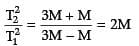⇒ T2 = √2T1 = 3√2

QUESTION: 39

A bar magnet having magnetic moment 0.4 JT–1 is placed in a uniform magnetic field of 0.16 T. The magnet is in stable equilibrium when the potential energy is

Solution:

In stable equilibrium, Potential energy U is defined as
U = 0MB
= - (0.4)(0.16)
= - 0.064J

QUESTION: 40

Two magnets are held together and are allowed to oscillate in the earth’s magnetic field with like poles together. 10 oscillations per minute are made but for unlike poles together only 4 oscillations per minutes are made. The ratio of magnetic moments is

Solution:

Here,
T1 = 60/10 = 6sec,
and T2= 60/4 = 15sec
M1/M2 = T22 + T12 / T22 - T12
= 29/21

QUESTION: 41

At a certain place, the angle of dip is 60°and horizontal component of earth’s magnetic field is             1 orested. The earth’s total magnetic field is

Solution:

We know that,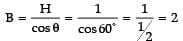QUESTION: 42

The susceptibility of annealed iron at saturation is 5000. The permeability is

Solution:

µr = 1 + χ
= 1 + 5000
= 5001
∵ μ = μrμ0 = 5001 (4π x 10-7)
= 6.28 × 10–3

QUESTION: 43

Two coils of self-inductances 9 mH and 4 mH are placed so close together that the effective flux in one coil is linked with other. The mutual inductance between these coils is

Solution:

Mutual inductance of two coils
M =  √M1M2
= √9mH x 4mH = 6mH

QUESTION: 44

Two solenoids of same cross-sectional area have their lengths and number of turns in the ratio of 1:3. The ratio of self-inductance of two solenoid is

Solution:

We know that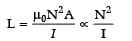⇒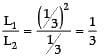QUESTION: 45

Assertion (A): A coulomb of charge is bigger than the charge on electron?
Reason (R): Electric charge is always quantized.

Solution:

Magnitude of charge on one electron, e = 1.6 × 10–19 Coulomb. According to the quantization of charge, charges of the particle (q) are integral multiple of charge of an electron/proton (e). i.e., q = ne. Therefore, number of charges in a coulomb,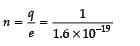= 0.625 x1019
Clearly shows that one coulomb of charge is bigger than the charge on electron and quantization of charge is the correct reason for it. So, both assertion and reason are true.

QUESTION: 46

Assertion (A): Potential difference between two points is depend on path.
Reason (R): Electric field is a conservative field.

Solution:

Potential difference between two points is defined as work done (W) in moving unit positive charge from one point A having potential VA to other point B having potential VB.
The work done is also explained as: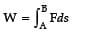We know that F = qE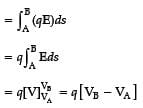As, electric field at a point (x,y), i.e.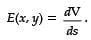It concludes that whatever path S is selected on moving charge from A to B, the integral will have only one value. It means potential difference between two points is independent of path. So, assertion is not true. Electric field is a conservative field i.e. line integral of an electric field along a closed path is zero.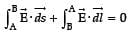So, assertion is incorrect and reason is correct.

QUESTION: 47

Assertion (A): Potentiometer measures the potential difference more accurately than a voltmeter.
Reason (R): There is no current drawn from external circuit in potentiometer.

Solution:

When a potential difference is measured using a voltmeter, voltmeter draws some current and when it is connected across the cell, there is a internal potential drop due to the internal resistance of the cell. Hence, measuring potential will not be accurate. In potentiometer, there is no current drawn from external circuit.
Therefore, both assertion and reason are correct and reason is also the correct explanation of A.

QUESTION: 48

Assertion (A): Earth’s magnetic field does not affect the functioning of a moving coil galvanometer.
Reason (R): Earth’s magnetic field is too weak.

Solution:

The coil of moving coil galvanometer is suspended in a very strong radial magnetic field. Earth’s magnetic field is too weak compared to that and hence its effect is negligible. So, assertion and reason both are true and the reason explains the assertion properly.

QUESTION: 49

Assertion (A): If an electron is not deflected when moving through a certain region of space, then the only possibility is that no magnetic field is present in that region.
Reason (R): Force on electron is directly proportional to the strength of the magnetic field.

Solution:

In absence of magnetic field, moving electron will not be deflected. This possibility is true. So, assertion is true.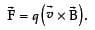So, force on electron is directly proportional to the strength of the magnetic field. So, reason is true. Reason properly explains the assertion.

QUESTION: 50

In the V-I graph for a given metallic wire at two different temperatures T1 and T2 are shown in figure. The relationship between T1 and T2 is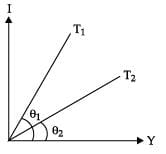Solution:

Given figure shows that slope of the line corresponding to T1 is greater than the slope of the line at T2, i.e., tan θ1 > tan θ2, which indicates that  T1 > T2.

QUESTION: 51

The given figure represents the material which is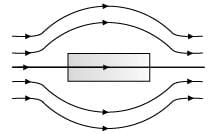Solution:

The given figure represents that magnetic field lines move away from the substance. The diamagnetic material has susceptibility c = 0, causing the repelling of magnetic field lines, Hence, given material is diamagnetic material

QUESTION: 52

CASE STUDY
Electric potential at a point is defined as the amount of work done in bringing a unit positive charge from infinity to that point.
So, electric potential = work done divided by the amount of charge moved.
Negative charges move from lower to higher potential. Positive charges move from higher to lower potential.
Charges gain energy while moving through a potential difference. If an electron is accelerated from rest through a potential difference of 1V, it gains 1 eV energy.
Formula of electric potential

Solution:

Electric potential at a point is defined using the following formula as V = W/Q, which states that the amount of work done in bringing a unit positive charge from infinity to that point is known as electric potential.

QUESTION: 53

An electron is accelerated through a potential difference of 7 volts. The energy gained by an electron will be

Solution:

Potential difference = 7 V
We know that
K.E. = 1/2mv2
here, 1/2mv2 = eV
K.E.   = e x 7V
K.E.   = 7eV

QUESTION: 54

Protons move from______ to ______ potential

Solution:

Proton has positive charge, in electric field positive charge moves from higher potential to lower potential.

QUESTION: 55

The amount of work done in bringing a charge of 10 mC from infinity to a point in an electric is 20 × 10–5 J. Electric potential at that point is

Solution:

Given that
Q = 10μC = 10 x 10-6C
W = 20 x 10-5 J
Since,
V = W/Q
= 20 x 10-5 / 20 x 10-6
= 20VUse Code STAYHOME200 and get INR 200 additional OFF Use Coupon Code